Humboldt State University
Mathematics Department Colloquium *
click on * to go to next section

September 24, 2017

Solving Polynomial Equations:
Visualization from Linear to Cubic,
from Rational to Complex Numbers

Martin Flashman
Professor Emeritus of Mathematics
Humboldt State University
Arcata, CA 95521
flashman@humboldt.edu
flashman.neocities.org

Abstract: From Euclid to Descartes, solving equations and visualization of relations between numbers have been a central theme of mathematics. In school mathematics these problems start formally in middle school and continue to the solution of the general quadratic equation where complex numbers are usually introduced. Cubic equations are more challenging and only special examples are treated until calculus  where applications of the intermediate value theorem are used to explain the solution of cubic equations. Rarely is any explicit formulation of the general solution of a cubic polynomial given or the nature of these solutions explored extensively.

Using some recent work with Geogebra on mapping diagrams, an alternative to graphs, Professor Flashman will provide visualizations for both the algebra used to solve linear and quadratic equations and the relation of quadratic and cubic equations to complex number solutions.

0. Preface:*

• Mapping Diagrams from A(lgebra) B(asics) to C(alculus) and D(ifferential) E(quation)s. A Reference and Resource Book on Function Visualizations Using Mapping Diagrams (In Development-Draft Version 8-2017)
• GeoGebra "Books"
Tom Edgar and N. Chris Meyer
The College Mathematics Journal
Vol. 48, No. 2 (March 2017), pp. 90-96
http://dx.doi.org/10.4169/college.math.j.48.2.90

I. What equations? *
What does it mean to solve an equation?
Find any and all numbers in the solution domain that make the equation a true statement when substituted for the equation's variable.
1.  Linear: $a_1 x+ a_0= 0$
Example: $3x -6 = 0.$
Solution: $x = 3$
Check!  OOOPS: $3\cdot 3-6=9-6=3 \ne 0$. Correct solution: $x=2$.
2. Quadratic: $a_2x^2 + a_1 x+ a_0= 0$
Example: $x^2 - 5x +6 = 0.$
Solution: $x = 3, x = 2$
Check!
3. Cubic:$a_3x^3 +a_2x^2 + a_1 x+ a_0= 0$
Example: $x^3 - 5x^2 +6x = 0.$
Solution: $x = 3, x = 2, x=0$
Check!

II. Solution Domains:
*
1. Natural Numbers:  $N=\{0 (?),1,2,... \}$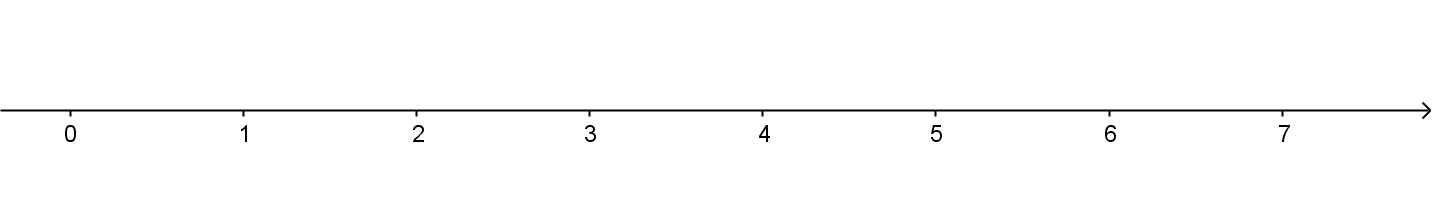Example: $3x -6 = 0.$
Solution: $3x -6 = 0$
$+6 = +6$
$3x= 6$
$\frac 13 \cdot (3x)=\frac 13 \cdot (6)$
$x = 3$
Check!
2. Integers: $Z=\{0,1,-1,2,-2,...\}$    *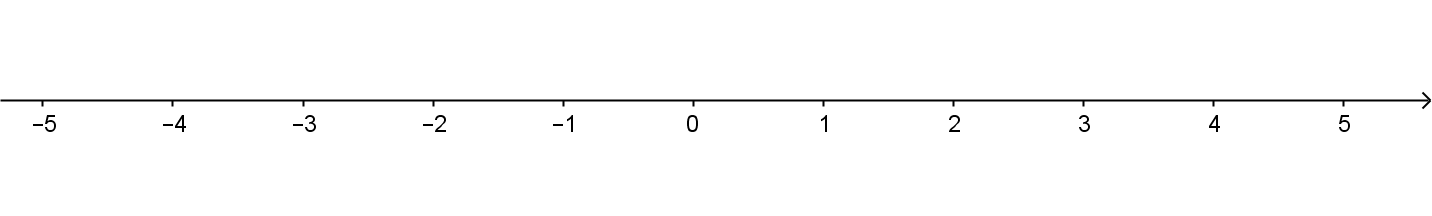Example: $3x +6 = 0$.
Solution: $3x +6 = 0$
$-6 = -6$
$3x= -6$
$\frac 13 \cdot (3x)=\frac 13 \cdot (-6)$
$x = -2$
Check!

3. Rational numbers: $Q=\{\frac pq, p,q \in Z, q\ne 0\}$. *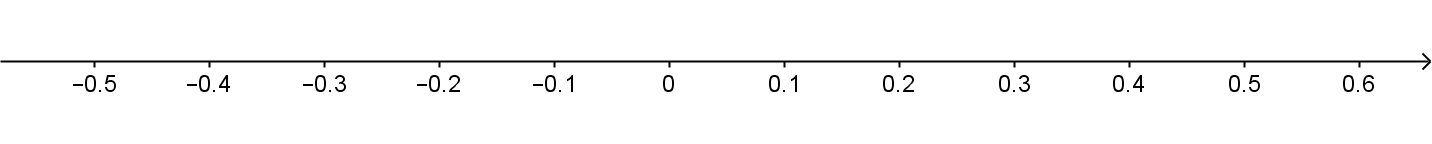Example: $3x - 4 = 0$.
Solution: $3x - 4 = 0$
$4=4$
$3x=4$
$\frac 13 \cdot (3x)=\frac 13 \cdot (4)$
$x = \frac 43$
Check!

4. Real Numbers: $R= \{ x: x= \pm ***.***..., (infinite)\ decimal \}$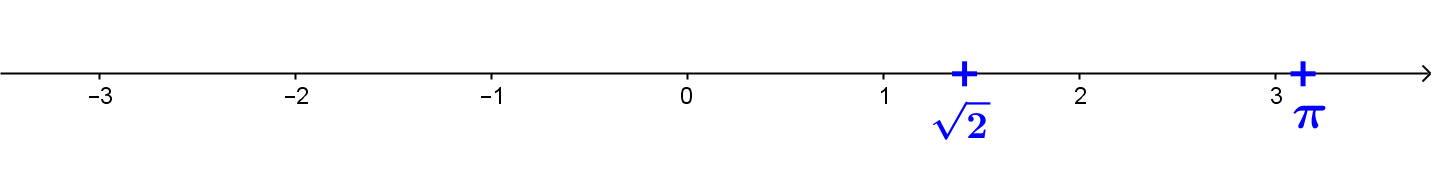Example: $x^2 - 2 = 0$.
Solution: $x = \sqrt 2, x= -\sqrt 2$
Check!

5. Complex Numbers: $C = \{a+bi: a,b \in R, i^2=-1\}$ *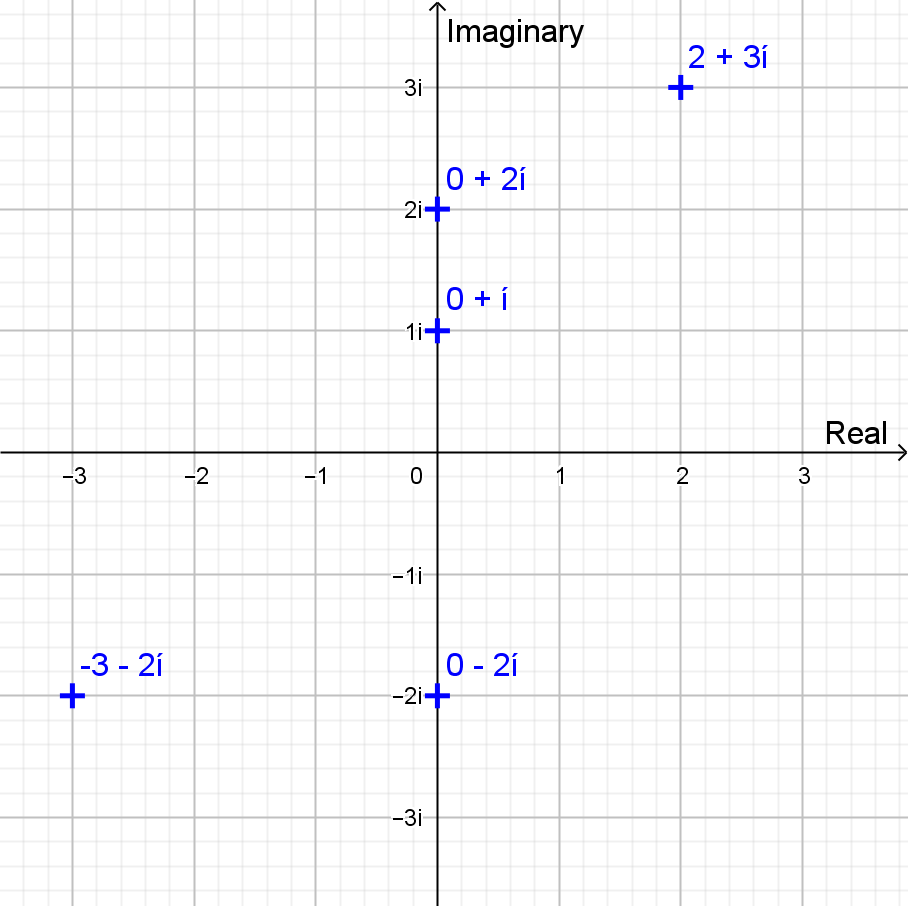Example: $x^2 + 4 = 0$.
Solution: $x = 2\sqrt {-1} = 2i, x= -2\sqrt {-1}=-2i ;\ i^2 = -1$
Check!

III Fundamental Theorem of Algebra *
If $a_nx^n+ ... +a_2x^2 + a_1 x+ a_0= 0$ is an equation with complex number coefficients then there are exactly n complex number solutions (allowing for possible repeated roots).

Or
Every non-constant single-variable polynomial with complex coefficients has at least one complex root. This includes polynomials with real coefficients, since every real number is a complex number with an imaginary part equal to zero.

First  stated in some form as early as about 1629 (Albert Girard), first proven (with a "gap") by  Carl Friedrich Gauss (1777-1855) in his Ph.D. Thesis (1799)  and a complete proof in its current form was given by Argand in 1816. [Just over 200 years ago!]
See Wikipedia:  https://en.wikipedia.org/wiki/Fundamental_theorem_of_algebra

IV Solving Equations Algebraically. *
[For clever solutions factoring is a key tool. FACT: If $a \times b = 0$ then either $a=0$ or $b=0$.]
1.  Linear: $a_1 x+ a_0= 0$
Example: $3x -6 = 0$.
$3(x-2)=0$
....
Solution: $x = 2$

2. Quadratic: $a_2x^2 + a_1 x+ a_0= 0$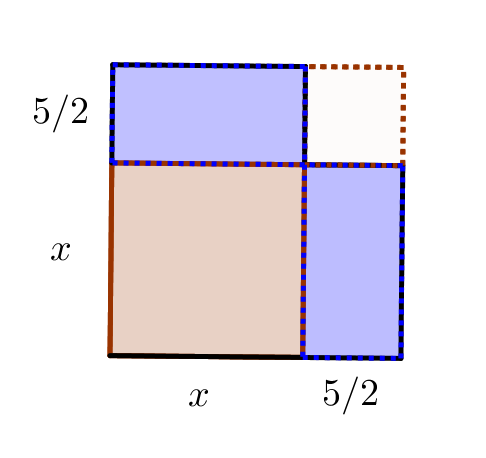Example: $x^2 + 5x +6 = 0$.   ["Completing the square."]

$(x+5/2)^2 - 1/4 = 0$

$[(x+5/2) + 1/2] [(x+5/2) - 1/2] = 0$
$(x+3)(x+2)=0$
...
Solution: $x = -3, x = -2$

3. Cubic:$a_3x^3 +a_2x^2 + a_1 x+ a_0= 0$
Example: $x^3 - 5x^2 +6x = 0$.
$x (x^2 - 5x +6) = 0$.
...

$x(x-2)(x-3)=0$
...
Solution: $x = 3, x = 2, x=0$

V. Visualizing Solving Equations.
*
• Polynomial Functions: $f(x) = a_nx^n+ ... +a_2x^2 + a_1 x+ a_0$ with real  (or complex) coefficients.
• Visualizing functions with tables and graphs.
• Visualizing functions with tables and mapping diagrams.

• Solving an equation $f(x) = 0$ visualized with table and graph.  [Look for graph of $f$ to cross X-axis.]
• Solving an equation $f(x) = 0$ visualized with table and mapping diagram. [Look for arrow on diagram to hit $0$ on target axis.]

• Main issues:
1. How do these visualizations connect to the algebra for solving an equation?
2. How do these visualizations connect to having complex numbers for solutions?

VI. Solving equations visualized with mapping diagrams *
• Linear and Quadratic Mapping Diagram Examples with GeoGebra
• Example: $2x +1 = 5$.
• Example: $x^2 - 5x +6 = 0$.
$(x-5/2)^2 - 1/4 = 0$
• Example: $2(x-3)^2 + 1 = 9$

VII. Complex Number Solutions to Real Polynomial Equations Visualized with Mapping Diagrams

• Quadratic Example: $x^2+Bx+C = 0$ *
• Solutions: $x = \frac {-B \pm \sqrt{B^2-4C}}{2}$
• Note connection of roots to value of (quadratic) discriminant $\Delta = B^2-4C$
• Particular examples to examine:
• Example: $x^2 -5x + 6= 0$
• Example: $x^2 -4x + 6= 0$

• Cubic Example: $x^3 +Bx^2+Cx+D=0$
• Replace $x$ by $x − B/3$:
$(x − B/3)^3 +B(x − B/3)^2+C(x − B/3)+D=0$
so the coefficient of $x^2$ in the resulting equation is $0$.
This changes the cubic to the so-called depressed cubic form $x^3 + px + q = 0$.
• Example: $x^3 - 5x^2 +6x = 0$; $B = -5, C=6$
Replace $x$ by $x + 5/3$:
$(x + 5/3)^3 -5(x + 5/3)^2+6(x + 5/3)=0$
$x^3 + 3\cdot 5/3 x^2 + 3\cdot 25/9x + 125/27 -5 x^2 - 50/3x - 125/9 +6x + 10=0$
$x^3 - 7 / 3 \cdot x + 20 / 27 =0$ ; $p=-7/3; q=20/27$.

• Solutions: Check Wikipedia: https://en.wikipedia.org/wiki/Cubic_function
• Note connection of roots to value of the related (cubic) discriminant
$\Delta = -(\frac q2)^2-(\frac p3)^3$
• Particular examples to explore: *
• Example: $x^3 - 1= 0$
• Example: $x^3 - x + 1= 0$
• Example: $x^3 -6x + 4= 0$
• Example: $x^3 -3x + 4= 0$

The End
Thanks!
Questions?